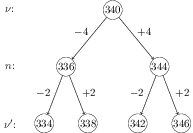# Beats

## Problems from IIT JEE

Problem (IIT JEE 2008): A vibrating string of certain length $l$ under a tension $T$ resonates with a mode corresponding to the first overtone (third harmonic) of an air column of length 75 cm inside a tube closed at one end. The string also generates $4$ beats per second when excited along with a tuning fork of frequency $n$. Now when the tension of the string is slightly increased the number of beats reduces to $2$ per second. Assuming the velocity of sound in air to be 340 m/s, the frequency $n$ of the tuning fork (in Hz) is,

1. 344
2. 336
3. 117.3
4. 109.3

Solution: The third harmonic in a pipe closed at one end occurs at a frequency, \begin{align} \nu=3v/(4L)=3\times 340/(4\times 0.75)={340}\;\mathrm{Hz}.\nonumber \end{align}Since string and closed pipe are in resonance, the frequency of string is $\nu={340}\;\mathrm{Hz}$. The tuning fork of frequency $n$ produces 4 beats with the string of frequency $\nu$. Thus, $n-\nu=4$ or $\nu-n=4$ i.e., $n={344}\;\mathrm{Hz}$ or $n={336}\;\mathrm{Hz}$. The frequency of string increases to $\nu^\prime$ with increase in tension $T$ as $\nu\propto \sqrt{T}$. Now tuning fork produces 2 beats. Thus, $n-\nu^\prime=2$ or $\nu^\prime-n=2$. The frequency $n={336}\;\mathrm{Hz}$ gives $\nu^\prime={334}\;\mathrm{Hz}$ or $\nu^\prime={338}\;\mathrm{Hz}$, which is not possible since $\nu^\prime>\nu$. The frequency $n={344}\;\mathrm{Hz}$ gives $\nu^\prime={342}\;\mathrm{Hz}$ or $\nu^\prime={346}\;\mathrm{Hz}$, which is possible.